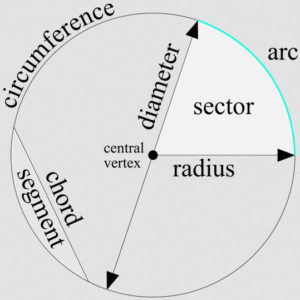# p5.js: Understanding circles

To understand how to code with circles in p5.js, it is necessary to have a foundation of knowledge about the parts of a circle and how they map to code.

The first mapping is the name. Circles are actually called ellipses in p5.js and are produced using the ellipse() function, that takes in four (or three) arguments. For example:

```// ellipse(x, y, w, h)
// h is optional, if left undefined then w will be used for both width and height
ellipse(50, 50, 80, 80);```

The first two arguments describe where to place the circle. They are the x and y coordinates of the circle’s origin point (center). The second two arguments are the width and height of the circle. The final argument, h, is optional. If left out, the ellipse will be a circle and w will be used for both width and height. If w and h values are different, then the ellipse is an oval, not a circle.

As can be seen in the following graphic, some of the parts of a circle are:

• central vertex (origin) – the center point of the circle
• circumference – the length of the outside line of the circle
• diameter – the total distance across the circle
• radius – half of the distance across the circle
• sector – a pie shaped piece of the circle
• chord – a line that cuts across the circle that doesn’t go through the origin
• segment – portions of the circle created by a chord
• arc – a section of the circumference
• tangent – a line outside the circle that intersects a point on the circleWhen working with ellipses, p5.js allows you to use either degrees or radians, but the default is radians. There is a function called angleMode() that allows you to switch either to degrees or radians. For example:

```// Change the mode to DEGREES
angleMode(DEGREES);

// Change the mode to RADIANS